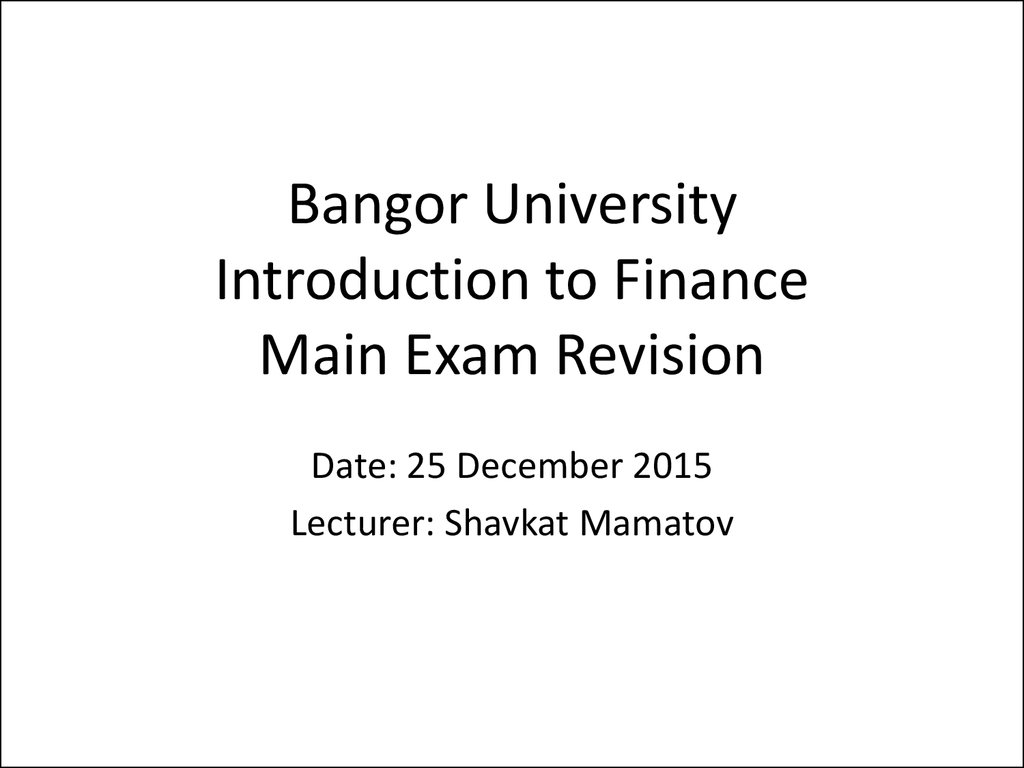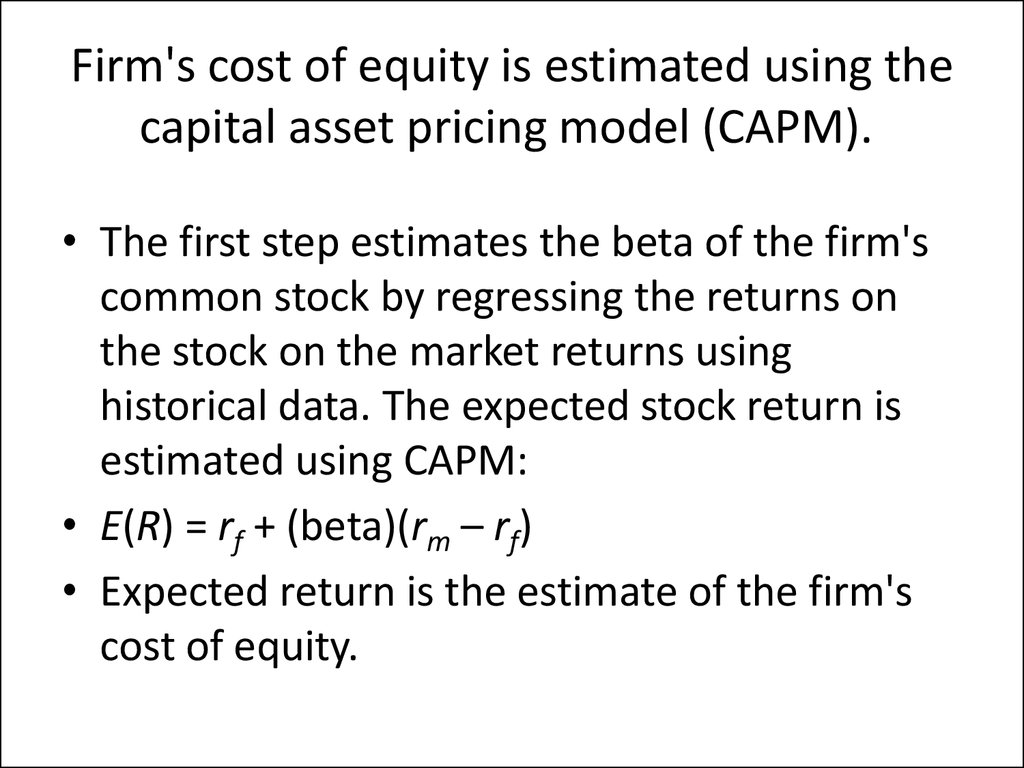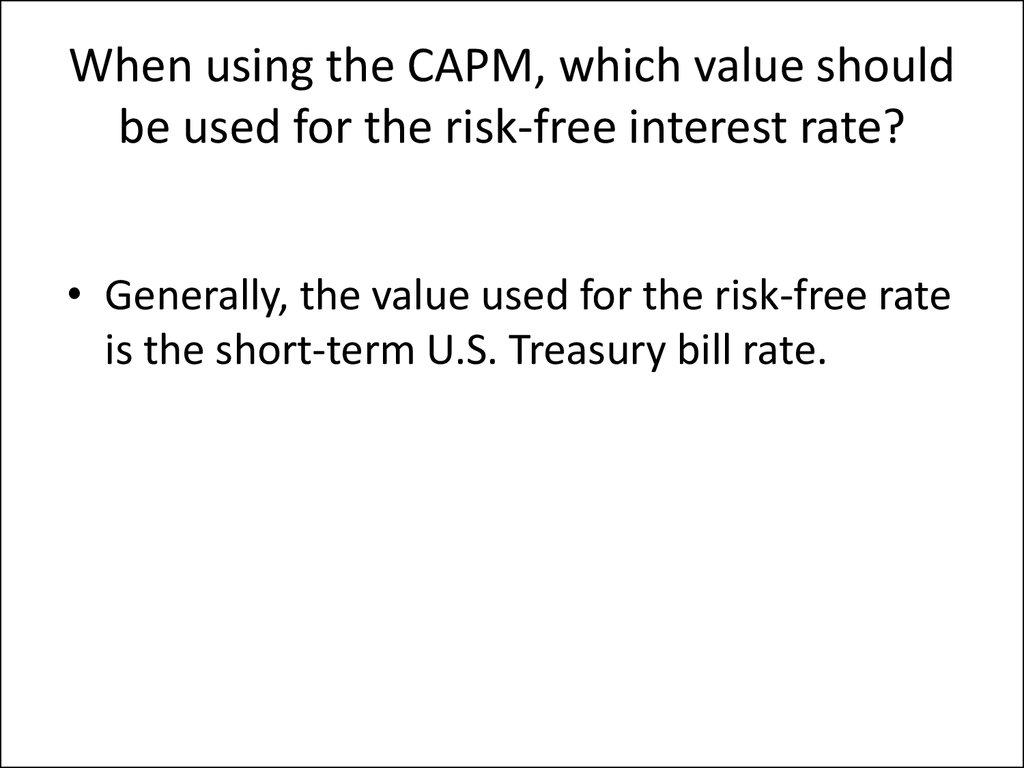# Introduction to finance

## 1. Bangor University Introduction to Finance Main Exam Revision

Date: 25 December 2015
Lecturer: Shavkat Mamatov

## 2. Firm's cost of equity is estimated using the capital asset pricing model (CAPM).

Firm's cost of equity is estimated using the
capital asset pricing model (CAPM).
• The first step estimates the beta of the firm's
common stock by regressing the returns on
the stock on the market returns using
historical data. The expected stock return is
estimated using CAPM:
• E(R) = rf + (beta)(rm – rf)
• Expected return is the estimate of the firm's
cost of equity.

## 3. When using the CAPM, which value should be used for the risk-free interest rate?

• Generally, the value used for the risk-free rate
is the short-term U.S. Treasury bill rate.

## 4. Factors that determine asset betas

• Asset betas are determined by the cyclical
nature of the cash flows. Generally, cyclical
firms have higher betas. Operating leverage
also affects the asset beta of a firm. Firms with
high fixed costs tend to have higher asset
betas.

## 5. The certainty equivalent approach to estimating the NPV of a project

• In the certainty equivalent approach, certainty
equivalent cash flows are discounted at the riskfree rate to calculate the NPV of a project. First,
risky cash flows have to be converted to
certainty-equivalent cash flows by using
individual risk factors. One advantage of this
method is that the risk adjustment is separated
from the time value of money. Conceptually this
is a more sensible method than the risk-adjusted
discount rate method. However, estimating
certainty equivalent cash flows could be
cumbersome.

## 6. The risk-adjusted discount rate approach to estimating the NPV of a project.

to estimating the NPV of a project.
• The risk-adjusted discount rate approach uses the
discount rate to adjust for both risk and the time
value of money
• The main advantage of this approach is simplicity.
Risky project cash flows are discounted using risk
adjusted discount rates (higher rates) to calculate
the NPV of a project.

## 7.

Stock Tiny and Stock Big have had the
following returns for the past three years: 12%, 10%, 32%; and 15%, 6%, 24%,
respectively. Calculate the covariance
between the two securities.
• E(Rtiny) = (-12 + 10 + 32)/3 = 10%;
E(Rbig) = (15 + 6 + 24)/3 = 15%;
Cov(Rting, Rbig) = [(-12 - 10)(15 - 15) + (10 10)(6 - 15) + (32 - 10)(24 - 15)]/(3 - 1) = 99.

## 8.

The market value of Ubez Corporation's
common stock is \$20 million, and the market
value of its risk-free debt is \$5 million. The
beta of the company's common stock is 1.25,
and the market risk premium is 8%. If the
Treasury bill rate is 5%, what is the company's
cost of capital? (Assume no taxes)
• rE = 5 + 1.25(8) = 15;
• rD = 5%.
Company cost of capital = 5% (5/25) + 15%
(20/25) = 1 + 12 = 13%.

## 9.

• The market value of Funny Corporation's
common stock is \$40 million and the market
value of its risk-free debt is \$60 million. The
beta of the company's common stock is 0.8,
and the expected market risk premium is 10%.
If the Treasury bill rate is 6%, what is the firm's
cost of capital? (Assume no taxes.)
• rE = 6 + 0.8(10) = 14%;
• rD = 5%;
• cost of capital = (60/100)(6%) + (40/100)
(14%) = 9.2%.

## 10.

• Company Alpen's historical returns for the
past three years are: 6%, 15%, and 15%.
Similarly, the market portfolio's returns were:
10%, 10%, and 16%. Calculate the beta for
Stock Alphine
• Beta = Cov(RA, RM)/Var(RM) = 0.75;
Cov(RB, RM) = [(6 - 12)(10 - 12) + (15 - 12)(10 12) + (15 - 12)(16 - 12)]/(3 - 1) = 9;
Var(RM) = [(10 - 12)^2 + (10 - 12)^2 + (16 12)^2]/(3 - 1) = 12.

## 11.

• The market portfolio's historical returns for
the past three years were 10%, 10%, and 16%.
Suppose the risk-free rate of return is 4%.

## 12.

• MahaCo. pays out 60% of its earnings as
dividends. Its return on equity is 15%. What is
the stable dividend growth rate for the firm?
• g = (1 - 0.60) × 15 = 6%.

## 13.

• Hardworking Co. just paid a dividend of \$2.00
per share. Analysts expect future dividends to
grow at 20% per year for the next four years
and then grow at 6% per year thereafter.
Calculate the expected dividend in year 5.
• Div5 = (2.00) × (1.20^4) × (1.06) = 4.40.

## 14.

• Tiotal Motor Company just paid a dividend of
\$1.40. Analysts expect its dividend to grow at
a rate of 18% for the next three years and
then a constant rate of 5% thereafter. What is
the expected dividend per share at the end of
year 5?
• D5 = (1.40) × (1.18^3) × (1.05^2) = 2.54

## 15.

• Sea Co. just paid a dividend of \$3 per share
out of earnings of \$5 per share. If its book
value per share is \$40, what is the expected
growth rate in dividends?
• g = (1 - 3/5)(5/40) = .05, or 5%.

## 16.

• The In-Tech Co. just paid a dividend of \$1 per
share. Analysts expect its dividend to grow at
25% per year for the next three years and
then 5% per year thereafter. If the required
rate of return on the stock is 18%, what is the
current value of the stock?
• P = (1.25/1.18) + (1.5625/1.18^2) +
(1.9531/1.18^3) + (2.0508/(0.18 0.05))/(1.18^3) = 12.97.

## 17.

• Sea Co. just paid a dividend of \$2 per share
out of earnings of \$4 per share. If its book
value per share is \$25 and its stock is currently
selling for \$40 per share, calculate the
required rate of return on the stock.
• g = (1 - 0.5)(4/25) = 0.08, or 8%;
• r = [(2 × 1.08)/40] + 0.08 = 13.4%.

## 18. Issues a company must take into consideration when determining the firms’ dividend policy

• In determining the firm’s dividend policy, two issues
are important: the dividend payout ratio and the
stability of the dividend payment over time.
• In this regard, the financial manager should consider
the investment opportunities available to the firm and
any preference that the company’s investors have for
dividend income or capital gains. Also, stock dividends,
stock splits, or stock repurchases can be used to
supplement or replace cash dividends.

## 19. The trade-offs in setting a firm’s dividend policy

• A. If a company pays a large dividend, it will thereby:
• 1. Have a low retention of profits within the firm.
• 2. Need to rely heavily on a new common stock issue for
equity financing.
• B. If a company pays a small dividend, it will thereby:
• 1. Have a high retention of profits within the firm.
• 2. Not need to rely heavily on a new common stock issue
for equity financing. The profits retained for reinvestment
will provide the needed equity financing.
• C. The importance of a firm’s dividend policy depends on
the impact of the dividend decision on the firm’s stock
price. That is, given a firm’s capital budgeting and
borrowing decisions, what is the impact of the firm’s
dividend policies on the stock price?

## 20. Real options associated with capital budgeting projects

• There are four types of real options. They are:
• options to expand.
• options to abandon.
• production options.
• timing options.
Options to expand provide a firm with flexibility to expand but do
not commit the firm to expand. Therefore, they add value to the
project. These are call options.
In many cases, the ability to terminate a project or abandon a
project adds flexibility to the project. This is useful when the project
fails to be profitable. Abandonment options are put options.

## 21.

• Production options provide a firm with additional flexibility
to alter inputs or processes. These have value when an
input becomes scarce and needs to be replaced with an
alternative. These production options add value to the
project.
In many cases, a positive-NPV project need not be
undertaken right away. It might be even more valuable if
undertaken in the future. The ability to postpone a project
also provides a firm with additional flexibility. These
options add value to the project.
A project may have multiple real options associated with it.
Many projects might become positive-NPV projects if the
real options associated with them are properly recognized
and evaluated.

## 22. Dividend Policy Matter to Stockholders?

• Three views about the importance of a firm’s dividend policy.
• View 1: Dividends do not matter.
• 1. Assume that the dividend decision does not change the firm’s
capital budgeting and financing decisions.
• 2. Assume perfect markets, which means:
• a. There are no brokerage commissions when investors buy and sell
stocks.
• b. New securities can be issued without incurring any flotation cost.
• c. There is no income tax, personal tax, or corporate tax.
• d. Information is free and equally available to all investors.
• e. There are no conflicts of interest between management and
stockholders.

## 23.

• 3. Under the foregoing assumptions, it may be shown that
the market price of a corporation’s common stock is
unchanged under different dividend policies. If the firm
increases the dividend to its stockholders, it has to offset
this increase by issuing new common stock in order to
finance the available investment opportunities. If on the
other hand, the firm reduces its dividend payment, it has
more funds available internally to finance future
investment projects. In either policy, the present value of
the resulting cash flows to be accrued to the current
investors is independent of the dividend policy. By varying
the dividend policy, only the type of return is affected
(capital gains versus dividend income), not the total return.

## 24.

• View 2: High dividends increase stock value.
• 1. Dividends are more predictable than capital gains because
management can control dividends, while they cannot dictate the
price of the stock. Thus, investors are less certain of receiving
income from capital gains than from dividend income. The
incremental risk associated with capital gains relative to dividend
income should, therefore, cause us to use a higher required rate in
discounting a dollar of capital gains than the rate used for
discounting a dollar of dividends. In so doing, we would give a
higher value to the dividend income than we would the capital
gains.
• Criticisms of view 2.
• a. Since the dividend policy has no impact on the volatility of the
company’s overall cash flows, it has no impact on the riskiness of
the firm.
• b. Increasing a firm’s dividend does not reduce the basic riskiness of
the stock; rather, if dividend payment requires management to
issue new stock, it only transfers risk and ownership from the
current owners to new owners.

## 25.

• View 3: Low dividends increase value.
• Stocks that allow us to defer taxes (low dividends-high
capital gains) will possibly sell at a premium relative to
stocks that require us to pay taxes currently (high
dividends-low capital gains). Only then will the two stocks
provide comparable after-tax returns which, suggests that a
policy to pay low dividends will result in a higher stock
price. That is, high dividends hurt investors, while low
dividends-high retention helps the firm’s investors. But
wait, then came 2003 and Congress again felt the need to
change the tax code as it pertained to both dividend
income and capital gains income. On May 28 President
Bush signed into law the “Jobs and Growth Tax Relief
Reconciliation Act of 2003.” Recall that part of the impetus
for this Act was the recession that commenced in 2001 and
the slow rate of payroll jobs creation that followed that
recession.

## 26. Sensitivity analysis as used for project analysis.

Sensitivity analysis as used for project analysis.
• By using sensitivity analysis one can determine
the factors which may have the largest impact on
project cash flows. For some projects, say, labor
costs might have a large impact on the cash
flows. That means that small changes in labor
costs will cause a large change in the cash flows
of the project. This helps the financial manager
and the project manager to focus on a few key
variables and take corrective or preventive
actions wherever possible. One drawback of
sensitivity analysis is that it gives ambiguous
results because variables are often interrelated.

## 27. The term price-earnings (P/E) ratio

• The P/E ratio is a widely used financial indicator, but
is also quite ambiguous. Generally, a high P/E ratio
indicates that the investors think a firm has good
growth potential. It is the ratio of current market
price and earnings of a stock. However, a high P/E
ratio can result from very low earnings

## 28. The assumptions associated with the constant dividend growth formula.

The assumptions associated with the constant
dividend growth formula.
• There are two important assumptions that are
necessary for the formula to work correctly. The first
assumption is that the expected growth rate of
dividends is constant. The second assumption is that
the discount rate is greater than the expected growth
rate in dividends.